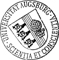## Ideal stream algebra

• We provide some mathematical properties of behaviours of systems, where the individual elements of a behaviour are modeled by ideals, ie. downward closed directed subsets of a suitable partial order. It is well-known that the associated ideal completion provides a simple way of constructing algebraic cpos. An ideal can be viewed as a set of consistent finite or compact approximations of an object which itself may be infinite. A special case is the domain of streams where the finite approximations are the finite prefixes of a stream. We introduce a special way of characterising behaviours through sets of relevant approximations. This is a generalisation of the technique we have used earlier for the case of streams. Given a subset P of a partially orderered set M, we define ide P as the set of downward closures of the directed subsets of P. We prove a number of distributivity and monotonicity laws for ide and related operators. They are the basis for correct refinement of specificationsWe provide some mathematical properties of behaviours of systems, where the individual elements of a behaviour are modeled by ideals, ie. downward closed directed subsets of a suitable partial order. It is well-known that the associated ideal completion provides a simple way of constructing algebraic cpos. An ideal can be viewed as a set of consistent finite or compact approximations of an object which itself may be infinite. A special case is the domain of streams where the finite approximations are the finite prefixes of a stream. We introduce a special way of characterising behaviours through sets of relevant approximations. This is a generalisation of the technique we have used earlier for the case of streams. Given a subset P of a partially orderered set M, we define ide P as the set of downward closures of the directed subsets of P. We prove a number of distributivity and monotonicity laws for ide and related operators. They are the basis for correct refinement of specifications into implementations. Various small examples illustrate that the operators lead to very concise while quite clear specifications. Finally, we give a characterisation of safety and liveness and generalise the Alpern/Schneider decomposition lemma to arbitrary domains. An extended example concerns the specification and transformational development of an asynchronous bounded queue.• 26230.pdfAuthor: Bernhard MöllerGND urn:nbn:de:bvb:384-opus4-262303 https://opus.bibliothek.uni-augsburg.de/opus4/26230 0302-9743OPAC Lecture Notes in Computer Science Springer Article English 1998 Universität Augsburg 2017/07/21 1546 69 116 https://doi.org/10.1007/3-540-49254-2_3 Fakultät für Angewandte Informatik Fakultät für Angewandte Informatik / Institut für Informatik Fakultät für Angewandte Informatik / Institut für Informatik / Professur für Programmiermethodik und Multimediale Informationssysteme 0 Informatik, Informationswissenschaft, allgemeine Werke / 00 Informatik, Wissen, Systeme / 004 Datenverarbeitung; InformatikDeutsches Urheberrecht